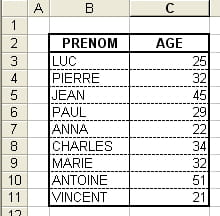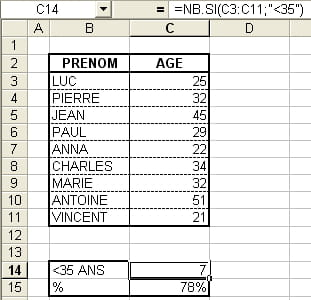0
Thanks

A few words of thanks would be greatly appreciated.

# Statistical functions: COUNTIF• Description : Used to count the number of cells in a range that meet a given criteria.

Syntax : COUNTIF(range,criteria)
Range is the range of cells where you wish to count the number of cells meeting the criteria specified in the formula.
criterion corresponds to the condition that a cell must fulfill to be counted by the formula.

Example : Consider a table representing a list of numbers. The objective is to know the percentage of people under 35 years.To describe the function, we perform the calculation in two steps:
Determining the number of people under 35 years.
Calculation of percentage.
Note : The calculation could be made ??directly in one step, the decomposition into two stages is made ??solely to illustrate the function.
We'll use the formula COUNTIF:

The criteria will be (for cell C14):
Range: C3:C11
Criterion: "<35"

The formula will be (for cell C14):
COUNTIF=(C3:C11,"<35")
To calculate the percentage of people under 35 years, we will combine the use of functions COUNTIF and COUNT.

The criteria used in the formula COUNTIF are the same as those specified in the example above.

The formula will be (for cell C15):
= (COUNTIF(C3:C11,"<35"))/(COUNT(C3:C11))

Explanation :
(COUNTIF(C3:C11,"<35")) gives us the number of people under 35 years.
(COUNT(C3:C11)) gives us the total number of people.
The relationship between the two gives us the proportion of people under 35 years in total.Note : the result of a calculation 0.777777777777778 C15 is equivalent to 7 / 9. To view 78%, just change the display format by selecting the cell percentage.
Two possibilities:
Select cell C15, click Format (in the menu bar) then click Cells. In the Number tab, choose the category Percentage.
More simply, you can also use the shortcut Percentage available in the toolbar
0
Thanks

A few words of thanks would be greatly appreciated.